Next: Scripted biases Up: Biasing and analysis methods Previous: Multidimensional histograms   Contents   Index

## Probability distribution-restraints

The histogramRestraint bias implements a continuous potential of many variables (or of a single high-dimensional variable) aimed at reproducing a one-dimensional statistical distribution that is provided by the user. The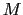variables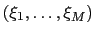are interpreted as multiple observations of a random variable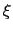with unknown probability distribution. The potential is minimized when the histogram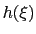, estimated as a sum of Gaussian functions centered at, is equal to the reference histogram:(13.26)(13.27)

When used in combination with a distancePairs multi-dimensional variable, this bias implements the refinement algorithm against ESR/DEER experiments published by Shen et al .

This bias behaves similarly to the histogram bias with the gatherVectorColvars option, with the important difference that all variables are gathered, resulting in a one-dimensional histogram. Future versions will include support for multi-dimensional histograms.

The list of options is as follows:

• name: see definition of name (biasing and analysis methods)
• colvars: see definition of colvars (biasing and analysis methods)
• outputEnergy: see definition of outputEnergy (biasing and analysis methods)

• lowerBoundary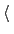Lower boundary of the colvar gridContext: histogramRestraint
Acceptable values: decimal
Description: Defines the lowest end of the interval where the reference distributionis defined. Exactly one value must be provided, because only one-dimensional histograms are supported by the current version.

• upperBoundary: analogous to lowerBoundary

• widthWidth of the colvar gridContext: histogramRestraint
Acceptable values: positive decimal
Description: Defines the spacing of the grid where the reference distributionis defined.

• gaussianSigmaStandard deviation of the approximating GaussianContext: histogramRestraint
Acceptable values: positive decimal
Default value: 2width
Description: Defines the parameterin eq. 13.28.

• forceConstantForce constant (kcal/mol)Context: histogramRestraint
Acceptable values: positive decimal
Default value: 1.0
Description: Defines the parameter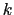in eq. 13.27.

• refHistogramReference histogramContext: histogramRestraint
Acceptable values: space-separated list ofpositive decimals
Description: Provides the values ofconsecutively. The mid-point convention is used, i.e. the first point that should be included is for= lowerBoundary+width/2. If the integral ofis not normalized to 1,is rescaled automatically before use.

• refHistogramFileReference histogramContext: histogramRestraint
Acceptable values: UNIX file name
Description: Provides the values ofas contents of the corresponding file (mutually exclusive with refHistogram). The format is that of a text file, with each line containing the space-separated values ofand. The same numerical conventions as refHistogram are used.

• writeHistogramPeriodically write the instantaneous histogramDescription: If on, the histogramis written every colvarsRestartFrequency steps to a file with the name outputName.name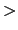.hist.datThis is useful to diagnose the convergence ofagainst.